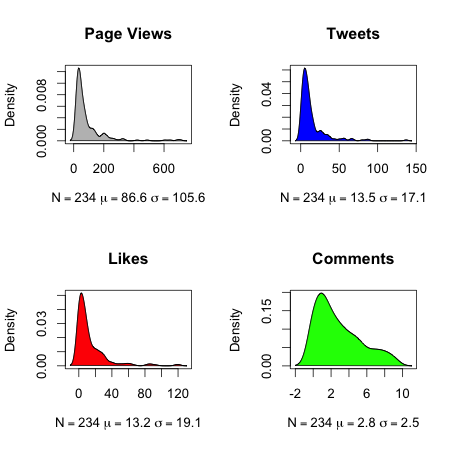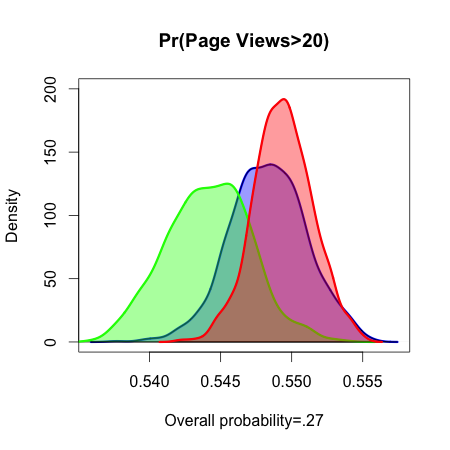In this post I continue the series begun here by asking, How do comments/tweets/likes correlate with page views? To answer this question I continue to use Anton Strezhnev's scrape of The Monkey Cage (TMC), which was described in the introductory post. I also use the Google Analytics (GA) data for TMC's top 2000 posts, which John Sides was kind enough to send me. (I'll discuss the data I collected soon.)

To combine the information from the two files, I wrote this script in Python that puts the dates and titles in the GA file into the form used in Strezhnev's data. Note that the titles in the GA file are truncated. I then matched the posts based on date and title in R using the substr() command to match on the first 10 characters. It is possible that this step introduced measurement error, so I am cautious about the results. In all, 234 of the 860 posts in Anton's data appeared in the top 2000 TMC posts, for an overall probability of .27. Here is how the number of page views, tweets, likes, and comments, are distributed over those 234 posts:

[caption id="attachment_744" align="aligncenter" width="450" caption="Reader Activity in 234 Monkey Cage Posts"][/caption]

Note that these data do NOT constitute a random sample--they are the 234 posts since May 2011 (the farthest that Anton went back) that are also in the top 2000 most popular Monkey Cage posts. Also, recall that TMC switched over to Wordpress hosting in early May, 2011, which complicated the title processing somewhat. The R code for the plot above is:

par(mfrow=c(2,2))

fancy_density = function(Variable, Name, Color) {
mu_var = round(mean(Variable, na.rm=T), digits=1)
sig_var = round(sd(Variable, na.rm=T), digits=1)
plot(density(Variable, na.rm=T),
main=Name,
xlab=bquote(paste(N==234,' ', mu==.(mu_var),' ', sigma==.(sig_var))))
polygon(density(Variable, na.rm=T), col=Color)
}

fancy_density(monkey1\$Visits, "Page Views", 'grey')
fancy_density(top2ktweets, "Tweets", 'blue')
fancy_density(top2klikes, "Likes", 'red')
fancy_density(top2kcomments, "Comments", 'green')

OK, enough with the setup--now that we know the distribution of the data, we can can begin modeling. If we wanted to model the relationship between the count of page views and the other three variables, we would use a negative binomial model. Let's make our task a bit simpler by just looking at the probability that the post is in the top 2000 (in other words, that it had over 20 direct page views, as distinct from visitors to the TMC's homepage).

[Skip this paragraph if not familiar with statistical analysis.] To do this, I ran a logistic regression of page views on tweets, likes, and comments. I then created 3 scenarios in which one of the variables is at its mean (rounded to the nearest integer) and the other two are at zero. For example, the tweets scenario (blue in the plot below) estimates the probability that a post is in the top 2000 given that it was tweeted 8 times, liked 0 times, and commented on 0 times. These scenarios account for uncertainty in the coefficient of interest using 1000 simulations. Note that the means used differ from those displayed with the density plots above since I used the mean for the whole sample, not just the most popular posts.

So how are tweets/likes/comments associated with the popularity of a post? As the plot below shows, an increased level of one form of reader activity predicts that a post is twice as likely as average to be ranked among the most popular--even while holding the other two levels of reader activity at zero.

[caption id="attachment_745" align="aligncenter" width="450" caption="Predicted probability that a TMC post is among the most popular, based on comments (green), tweets (blue), and likes (red)."][/caption]

As the plot shows, likes have the closest correlation with the popularity of posts, followed closely by tweets (indicated by the overlap of the probability densities). Comments have a similar but slightly lower association, but this could be due to the fact that comments fall within a much narrower range than tweets and likes (see above). This association is fortunate because it indicates that comments can serve as a suitable proxy for the popularity of posts when we consider data from the other websites, for which page view and tweet/like data have not been collected. Next time, we will consider how the individual attributes of a post (length, images, etc.) are associated with its popularity.